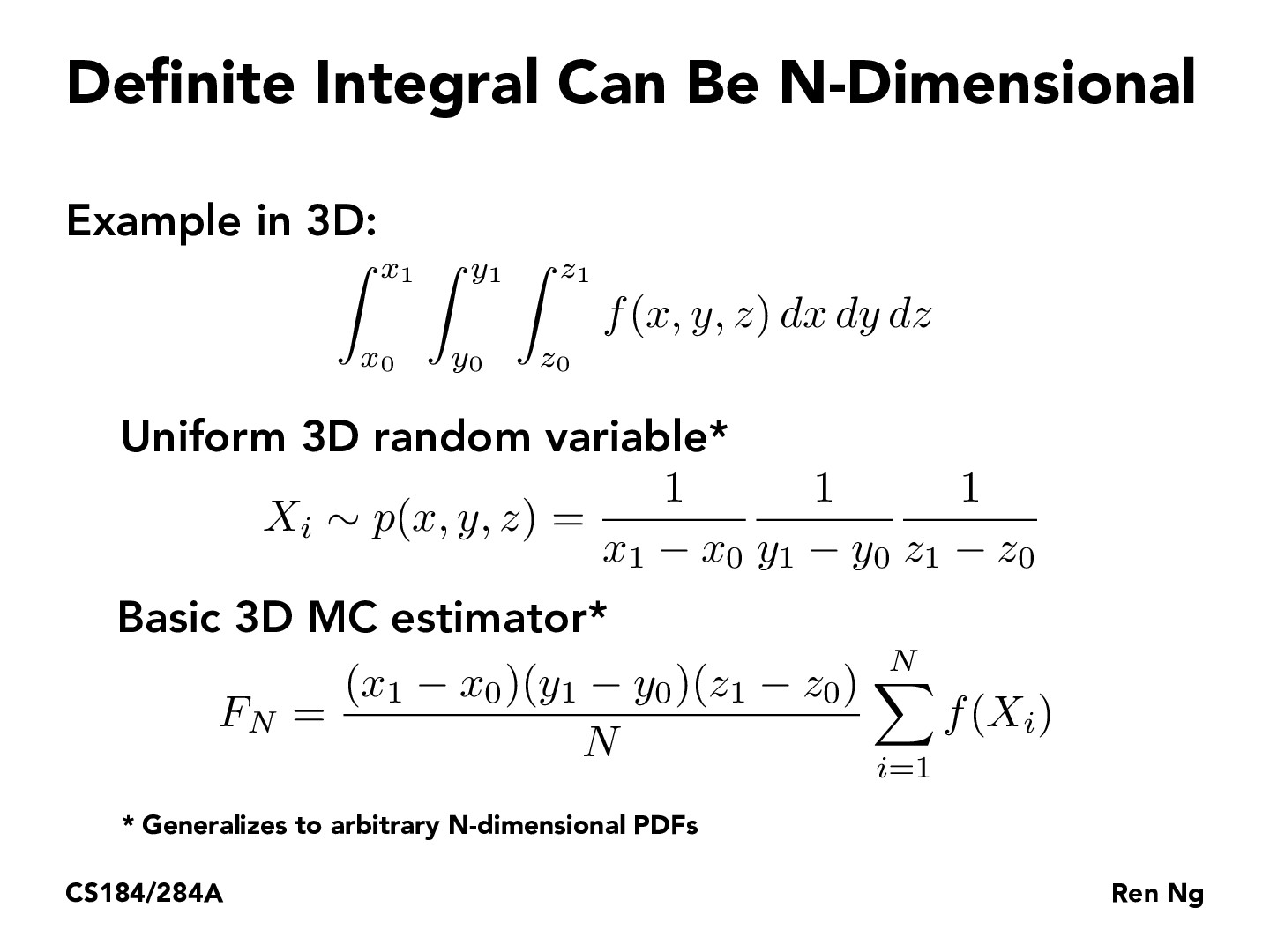Lecture 12: Monte Carlo Integration (29)rileylyman184

Something interesting I noticed is that calculating the monte carlo estimator for a 1D function is equivalent to finding the area of a 2D rectangle and finding the estimator for a 3D function is equivalent to finding the volume of a 4D hypercube. Generalizing this, we can see that integrating any n dimensional function will be equivalent to finding the volume of a n+1 dimensional hypercube. This is because the n axes of the function are like the width and length, etc, of the cube, and the actual value of the function is the last missing piece that adds another dimension.

You must be enrolled in the course to comment